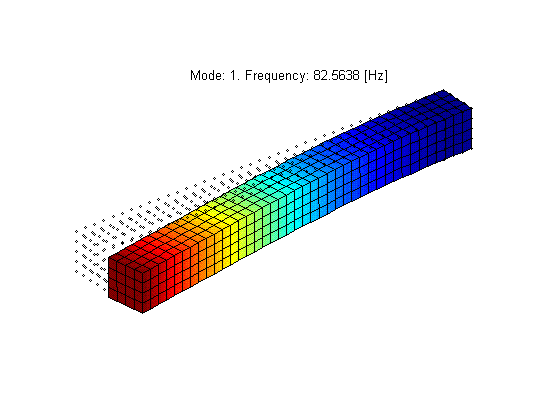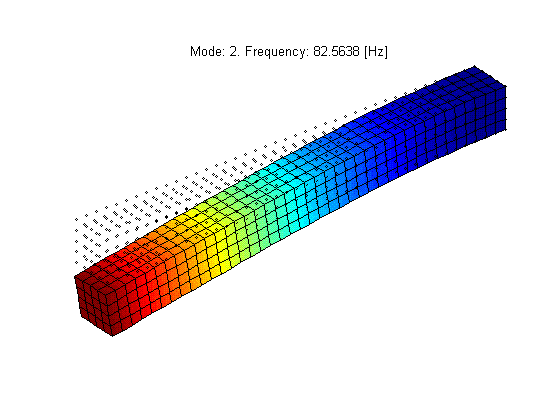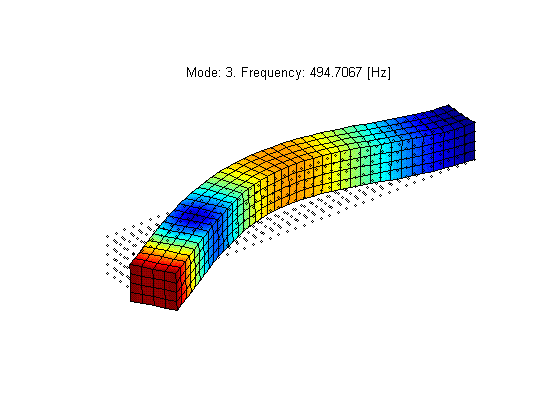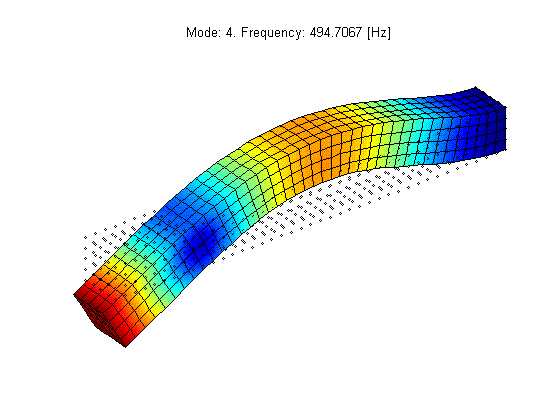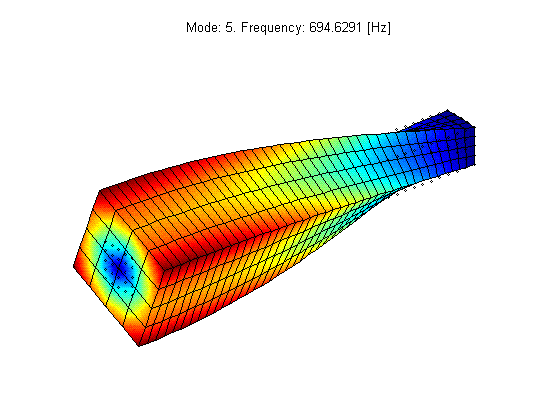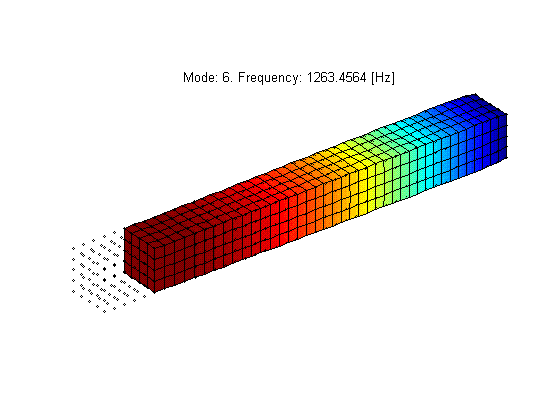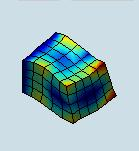Date:
 ``` Static and modal analysis of a steel beam (input model provided by: Andreas Hoffmann). Dimensions: length = 1.0 m, width = 0.1 m, height = 0.1 m. Material properties: E = 2e11 Pa, ρ = 7890 kg/m3, ν = 0.3. Load: vertical at free-end -10 N. Mesh size: 0.025 m. ``` ``` Static analysis: ```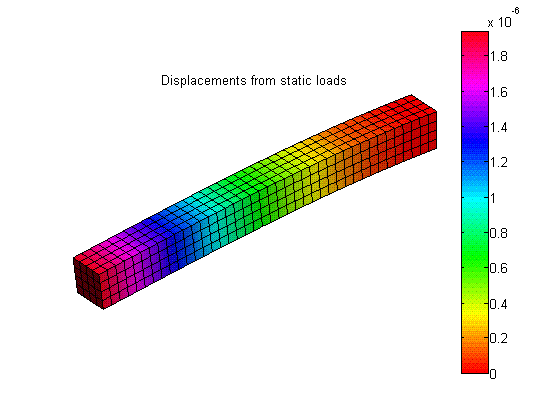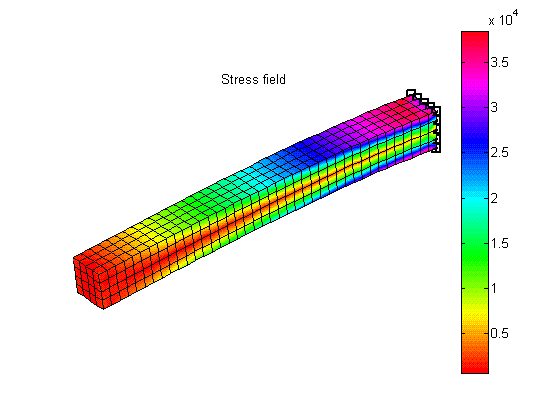``` Modal shapes: ```